# Machine Learning 101 – Overfitting and Underfitting

Remember that in the previous lecture (Lecture 6), we  discuss polynomial curve fitting. We kind of saw that the relationship in a dataset can be represented using a polynomial of this form: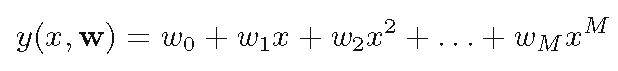Just assume that this is any polynomial of order, M. We want to understand want happens when the order gradually increases from 0 to say 13.

Also assume that the w terms are constant.

Remember our goal is to ensure that this polynomial fits the dataset. That is, after plotting the dataset as a scatter plot, then we fit this polynomial through it. We’ll then cover the following:

#### 1. How M relates to E

Also recall that we would like to minimize the error term E(x, w*). This is so that the difference between predicted values and the actual values is very small.

Now as M (the order of the polynomial) increases, the error, E decreases. This also means that the model complexity increases since we have higher order polynomial.

Similarly, when M is low, the complexity of the model reduces, meaning that the model becomes kind of simple. (I recommend you take some time to understand this relationship).

So it then appear that to reduce the error E to the barest minimum, or even zero, we simply keep increasing M. But there a problem.

Let’s now see what happens when M is too low and when M is too high.

#### 2. If M is very low (Underfitting)

As mentioned, if M is very low, then  polynomial is will not be able to properly model the relationship between the variable.  So the following will be true:

• the model (polynomial) will not give a good fit to the dataset
• the model is simple and easy to manipulate
• the error is high

You can see how the polynomial looks for values of M =1 and M = 3 in the figure below.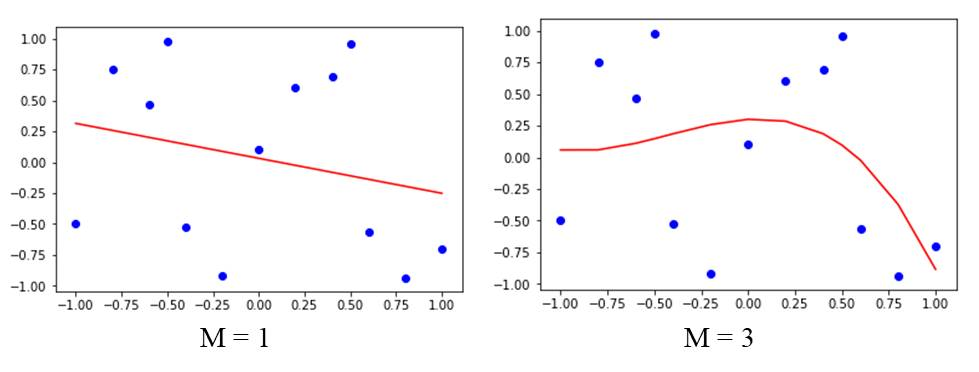You can see that the model does not  fit the given dataset properly. This problem is called underfitting.

#### 3. If M is very high (Overfitting)

On the other hand, if M is very high, then the following will be true:

• the model will fit the dataset very closely or even match every point in the dataset
• the model’s complexity will increase
• the error also becomes high (it decreases, then increases again)

I have generated the curve for values of M = 11 and M = 12 as shown below.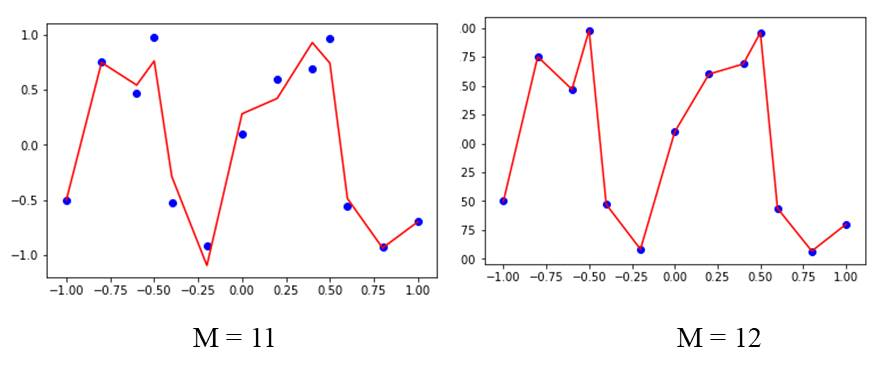In this case of M = 11 and M =12, you can see that the model fits the data very closely. There are two problems with this:

• first, the model becomes too complex
• second, the model is not able to generalize (predict new values) properly

So this is the problem of overfitting.

Therefore, we need to find a trade-off between the too extremes we just discussed. This trade-off has a special name in Machine Learning. It is called Bias-Variance Trade-off. It would be discussed further is subsequent lectures. Bias-Variance Trade-off Video.

At the trade-off point, the error is minimum.

I have generated the plot for values of M = 6 and M = 7 as shown below: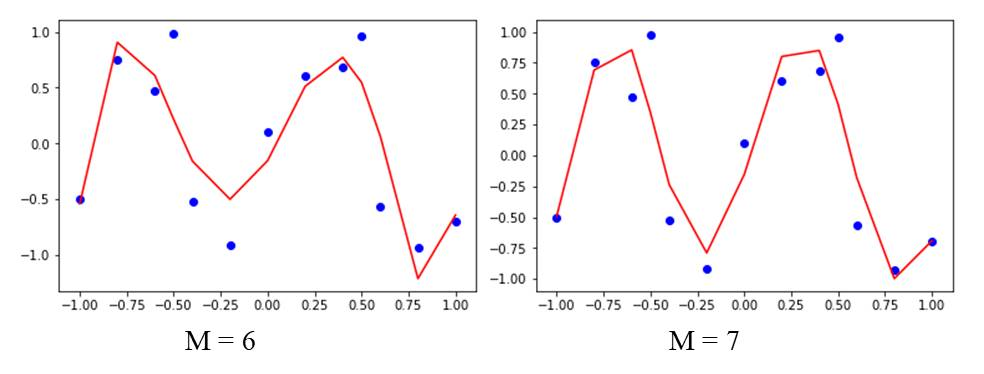#### 5. Hands on in Python

Now I would like to play around with this in Python. The complete code is given below. Try to adjust the values of M gradually from 0 to 5 and see how it affects the graph.

The complete code is given below.

You can adjust M by changing the degree.

Python Code here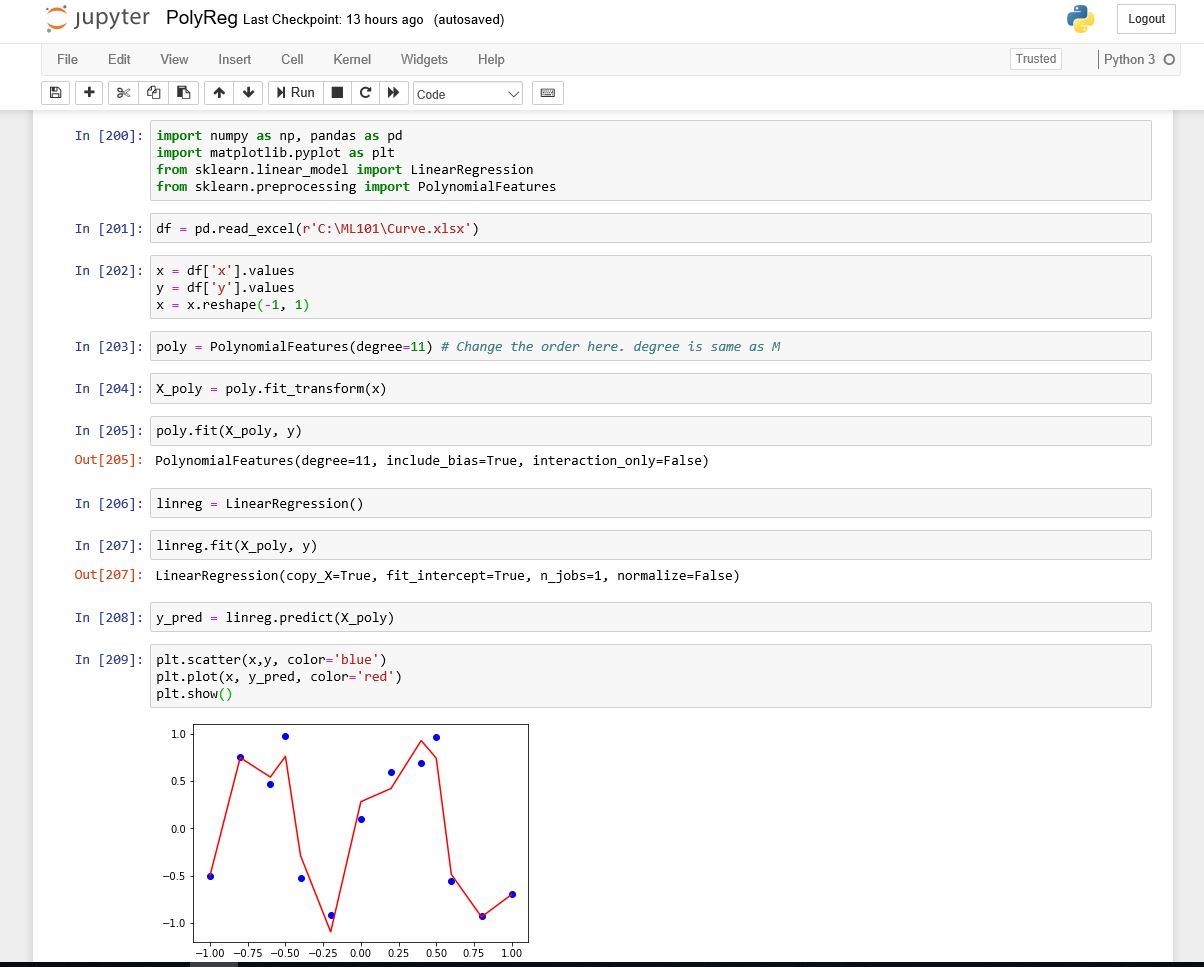#### kindsonthegenius

Kindson Munonye is currently completing his doctoral program in Software Engineering in Budapest University of Technology and Economics

View all posts by kindsonthegenius →